# Test: Inequalities

## 40 Questions MCQ Test Quantitative Aptitude for CA CPT | Test: Inequalities

Description
Attempt Test: Inequalities | 40 questions in 40 minutes | Mock test for CA Foundation preparation | Free important questions MCQ to study Quantitative Aptitude for CA CPT for CA Foundation Exam | Download free PDF with solutions
QUESTION: 1

Solution:
QUESTION: 2

### Find  the range of real values of x satisfying the inequalities 3x-2 > 7 and 4x - 13 > 15

Solution:

3x - 2 > 7 => 3x > 9 => x > 3
and 4x - 13 > 15 => 4x > 28 => x > 7, 7 is greater than 3.
Therefore, x > 7.

QUESTION: 3

### The shaded region represents: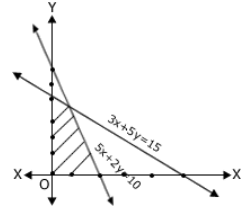Solution:
QUESTION: 4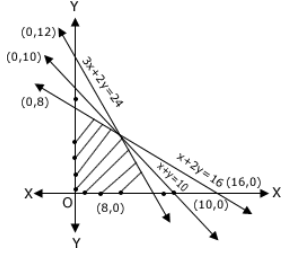Solution:

The shaded region is below the lines 3x + 2y = 24, x+2y = 16, x+y = 10

and above the lines x = 0 , y = 0

QUESTION: 5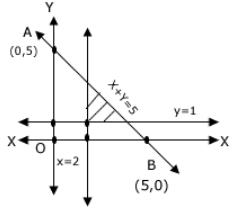Solution:
QUESTION: 6

On the average, experienced person does 5 units of work while a fresh one 3 units work daily but the employer have to maintain the output of at least 30 units of work per day. The situation can be expressed as.

Solution:
QUESTION: 7

The Linear relationship between two variables in an inequality :

Solution:
QUESTION: 8

The solution of the inequality 8x + 6 < 12x + 14 is

Solution:
QUESTION: 9

The union forbids employer to employ less than two experienced person (x) to each, fresh person (y). This situation can be expressed as:

Solution:
QUESTION: 10

Solution space of the inequalities 2x + y ≤ 10 and x - 7 ≤ 5:
(i) includes the origin.
(ii) includes the point (4,3)
which one is correct?

Solution:
QUESTION: 11

The graph of linear in equalities 7x + 9y ≤ 63, x + y ≥1, 0 ≤ x ≤ 6 and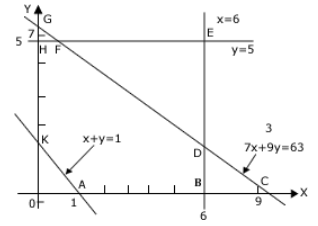Solution:
QUESTION: 12

On an average, experienced person does 5 units of work while a fresh person does 3 units of work daily but the employer has to maintain the output of atleast 30 units of work per day The situation can be expressed as.

Solution:
QUESTION: 13

The common region satisfying the set of inequalities x≥ 0, y ≥ 0 , L1 : x+ y ≤ 5, L2 : x + 2y ≤ 8 and L3: 4x+ 3y ≥ 12 is indicated by

Solution:
QUESTION: 14

By solving the inequality 3(a - 6) < 4 + a, the answer will be

Solution:
QUESTION: 15

The region indicated by the shading in the graph is expressed by inequalities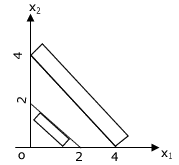Solution:
QUESTION: 16

On solving the inequalities 6x+ y ≥ 18, x+4y ≥ 12, 2x+y ≥ 10, we get following situation

Solution:
QUESTION: 17

A car manufacturing company manufactures cars of two types A and B. Model A requires 150 man-hours for assembling, 50 man-hours for painting and 10 man-hours for checking and testing. Model B requires 60 man-hours for assembling, 40 man-hours for painting and 20 man-hours for checking and testing. There are available 30 thousand man-hours for assembling, 13 thousand man-hours for checking and testing. Express the above situation using linear inequalities. Let the company manufacture x units of type A model of car and y units type B model of car. Then, the inequalities are:

Solution:
QUESTION: 18

The set of inequalities L1 : x1 + x2 ? 12, L2: 5x1 + 2x2 ? 50, L3 : x1 + 3x2 ? 30, x1 ? 0 and x2 ? 0 is represented by

Solution:

QUESTION: 19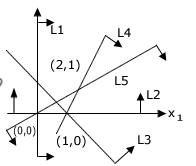The common region indicated on the graph is expressed by the set of five inequalities

Solution:
QUESTION: 20

On solving the inequalities 6x + y ≥ 18, x + 4y ≥ 12, 2x + y≤ 10, we get the following situation:

Solution:
QUESTION: 21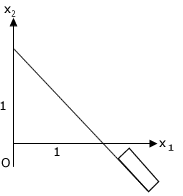The region is expressed as

Solution:
QUESTION: 22

Graphs of Inequations are drawn below: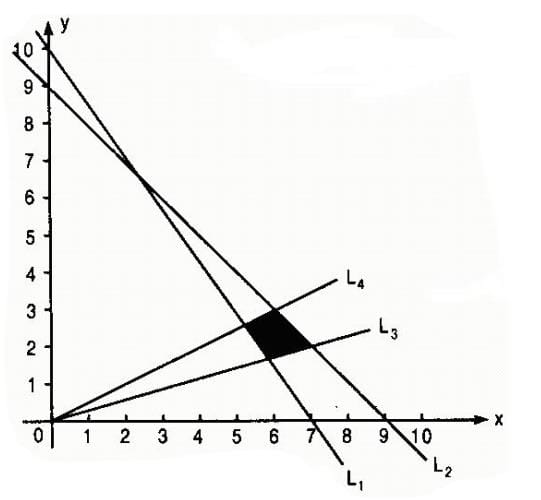L1 : 5x + 3y = 30 L2 : x+y = 9 L3 y = x/3 L4: y = x/2
The common region (shaded part) shown in the diagram refers to the inequalities:

Solution:
QUESTION: 23

A firm makes two types of products: Type A and Type B. The profit on product A is Rs. 20 each and that on product B is Rs. 30 each. Both types are processed on three machines M1, M2 and M3. The time required in hour by each product and total time available in hours per week on each machine are asa follows:
Machine   Product A   Product B   Available Time
M1               3                 3                36
M2               5                 2                50
M3               2                 6                60
The constraints can be formulated taking x1 = number of units A and x2 = number of unit of B as

Solution:
QUESTION: 24

The graph to express the inequality x + y ≤ 9 is

Solution:
QUESTION: 25

The rules and regulations demand that the employer should employ not more than 5 experienced hands to 1 fresh one and this fact be expressed as

Solution:
QUESTION: 26

The graph to express the inequality 5x + 3y ≥ 30 is

Solution:
QUESTION: 27

The common region satisfied by the inequalities L1 : 3x + y ≥ 6, L2 : x + y ≥ 4, L3: x + 3y ≥ 6, and L4: x + y ≤ 6 is indicated by

Solution:
QUESTION: 28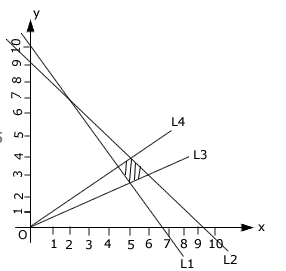L1 : 5x + 3y = 30 L2 : x+y = 9 L3 : y = x/3 L4 : y = x/2
The common region (shaded part) shown in the diagram refers to

Solution:
QUESTION: 29

The region indicated by the shading in the graph is expressed by inequalities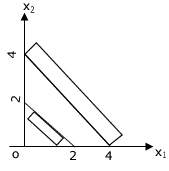Solution:
QUESTION: 30

Graph of the inequations are below: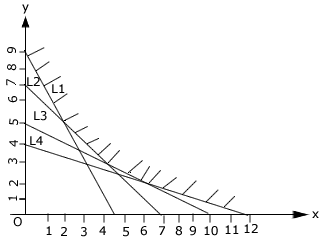L1 : 2x + y = 9 L2 : x + y = 7 L3 : x + 2y = 10 L4: x + 3y = 12
The coomon region (shaded part) indicated on the diagram is expressed by the set of inequalities

Solution:
QUESTION: 31

The union however forbids forbide him to employ less than 2 experiencedd person to each fresh person. This situation can be expressed as

Solution:
QUESTION: 32

A dietitian wishes to mix together two kinds of food so that the vitamin content of the mixture is at least 9 units of vitamin A, 7 units of vitamin B, 10 units of vitamin C and 12 units of vitamin D. The vitamin content per Kg. of each food is shown below.
Food I : A = 2 , B = 1, C = 1, D = 2
Foof II : A = 1,  B = 1, C = 2 ,D = 3
Assuming x units of food I is to be mixed with y units of food II the situation can be expressed as

Solution:
QUESTION: 33

An employer recruits experienced (x) and fresh workmen (y) for his firm under the condition that he cannot employ more than 9 people. X and Y can be related by the inequality

Solution:
QUESTION: 34

Mary scored 200 marks in three tests. If average score of 60 is required then the lowest marks she must score for fourth test are

Solution:
QUESTION: 35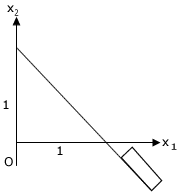The region is expressed as

Solution:
QUESTION: 36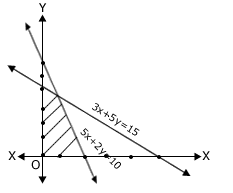Solution:
QUESTION: 37

The union forbids employer to employ less than two experienced person (x) to each, fresh person (y). This situation can be expressed as:

Solution:
QUESTION: 38

The Linear relationship between two variables in an inequality :

Solution:
QUESTION: 39

On solving the inequalities 6x + y ≥ 18, x + 4y ≥ 12, 2x + y≤ 10, we get the following situation:

Solution:
QUESTION: 40

On solving the inequalities 6x+ y ≥ 18, x+4y ≥ 12, 2x+y ≥ 10, we get following situation

Solution:Use Code STAYHOME200 and get INR 200 additional OFF Use Coupon Code